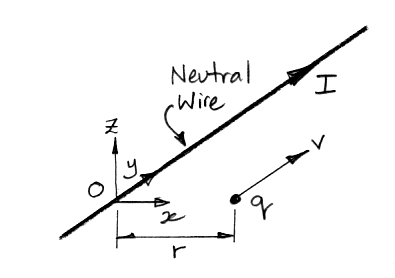# Relativistic Electromagnetism

© 2021 Kevan Hashemi, Brandeis University

## Contents

Introduction
Current-Carrying Wire and Moving Charge

## Introduction

By means of a series of problems, we investigate how the manner in which we calculate the forces generated by the electric and magnetic fields are affected by the movement of charges.

## Current-Carrying Wire and Moving Charge

[12-JAN-21] A particle with electric charge q is at a radius r from an infinite, straight wire carrying a current I. We choose the frame of reference, O, in which the wire is stationary, and place our coordinate origin on the wire so that the particle is at x = r and the y-axis is parallel to the wire and in the direction of the current.Figure: Charged Particle Moving Parallel to a Current-Carrying Wire. The wire is stationary and neutral.

When we say the wire is neutral, we mean it has no net electric charge. When we say the wire carries a current I we mean that I coulombs of electrons move in the opposite direction to the current through any cross-section of the wire each second.

What is the magnitude and direction of the magnetic field, B, at the location of the charged particle?

What is the magnitude and direction of the force exerted by the magnetic field upon the charged particle due to its motion?